>>>  Go to Pro/E Tips Index  >>>
>>>  Page to Previous Pro/E Tip  >>>

NOVEMBER 1998

Power Tools:  Variable Section Sweep

Pro/ENGINEER has several nice features for creating complex geometry, however, to get the most from them you sometimes need to know a few tricks.  Here are 5 quick tips to use with Variable Section Sweeps to make them a little more user friendly.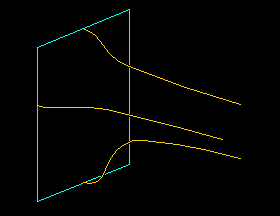FIGURE 1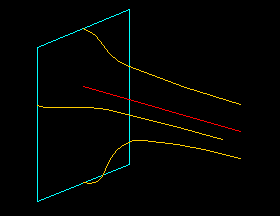FIGURE 2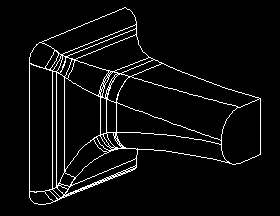FIGURE 3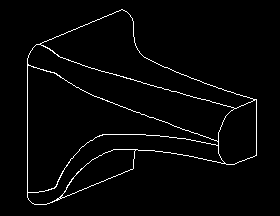FIGURE 4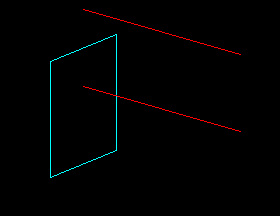FIGURE 5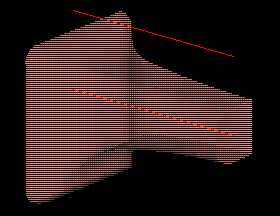FIGURE 6
Variable Section Sweeps require at least 2 trajectories -- 1 to define the path, and 2 to define the orientation of the section as it follows the path.  Additional trajectories may be added to further define how the section will vary from beginning to end.

Figure 1 is an example of 3 trajectories and a section to define a new variable section sweep.  By using the Pivot Dir option, the desired protrusion can be attained, but sometimes the result is not as expected.

TIP:   Control the section using the Normal To Traj option then add a first trajectory to guide the section as you desire.

The red curve of Figure 2 shows the first trajectory for our example.  It was sketched "on the fly".  The other 3 trajectories were then selected to control the section.

Note:  This example uses a straight trajectory sketched "on the fly", but just about any curve will work.

TIP:   Be sure the first trajectory adequately describes the desired path.  The section will follow this path and vary as it goes.  Be sure the path you define will not cause the section to overlap or fold on itself.  Failures and "funny" ripples are often caused by a poorly placed first trajectory.

Next, understand the effect of trajectories on your final geometry.  If the trajectories of Figure 2 are created from arcs, the resulting protrusion will have tangent though curvature discontinuous surfaces.  This is seen by the tangent lines appearing on the solid.  The effect of this may be similar to the protrusion of Figure 3, where rounds have been added to show the compounding effects of additional geometry added later.

Note:  Surfaces such as these are not intrinsically bad, however, when they are small like those of Figure 3, they can cause problems in later geometry, and create headaches with FEA and CAM.

TIP:   Use composite curves to create continuous surfaces.

The protrusion of Figure 4 was created using the curves of Figure 2, but the 3 orange curves were changed to composites. Notice the tangent lines of the sweep are gone, and the added rounds create single surfaces resulting in "cleaner" geometry.

Another approach to the Variable Section Sweep is control by equation or graph.   Here, the sweep needs only 2 trajectories. The first is the same as above.  The second defines only the orientation of the section so it can simply be parallel as in Figure 5.

TIP:   When controlling a sweep by equation or graph, place the X-trajectory away from the section to avoid undesired sketcher assumptions.

Section dimensions are then controlled by relation equations or by graph features.

The resulting solid with highlighted trajectories is shown in Figure 6.
(Rounds have been added for consistency with the above pictures.)

Note:  The above discussion about using composite curves applies to the use of graphs also.  If multiple entities are used in the graph, the resulting geometry will have tangency lines.

TIP:   To maximize flexibility, you can mix and match the above techniques -- using trajectories, equations, and graphs.  In this way you can create truly astounding geometry.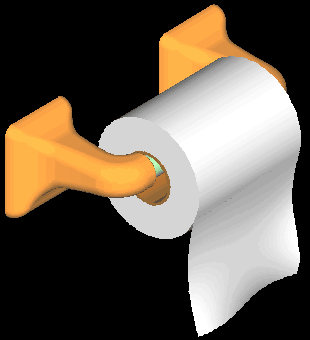From our example above, we just need to ... add a protrusion,      a few rounds,           a couple other parts -- and we're are ready to Roll.     Good Luck with all your Variable Section Sweeps!

.

SYNTHESIS ENGINEERING SERVICES INC. : (719) 380-1122